# 【论文阅读】Deep High-Resolution Representation Learning for Visual Recognition

## HRNet

Posted by x-jeff on May 13, 2023

# 1.INTRODUCTION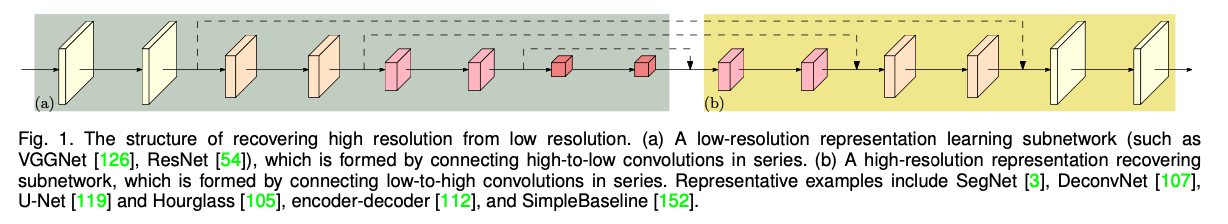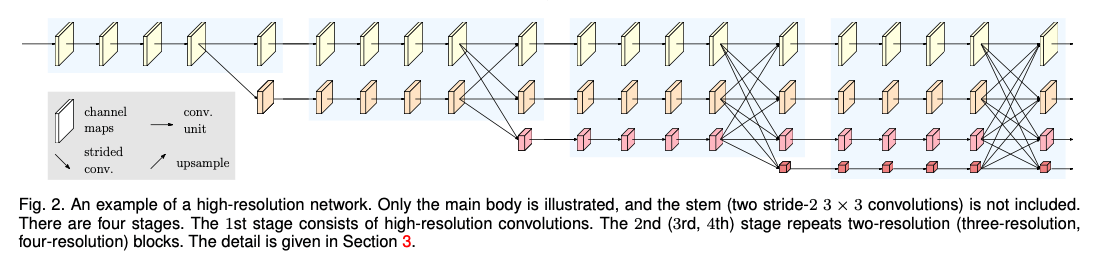# 2.RELATED WORK

👉Learning low-resolution representations.

👉Recovering high-resolution representations.

👉Maintaining high-resolution representations.

👉Multi-scale fusion.

👉Our approach.

# 3.HIGH-RESOLUTION NETWORKS

## 3.1.Parallel Multi-Resolution Convolutions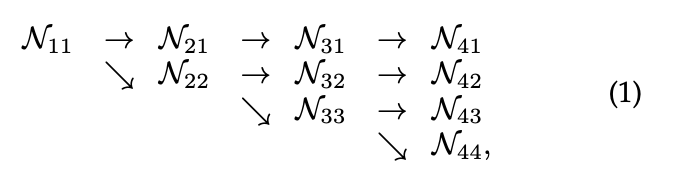## 3.2.Repeated Multi-Resolution Fusions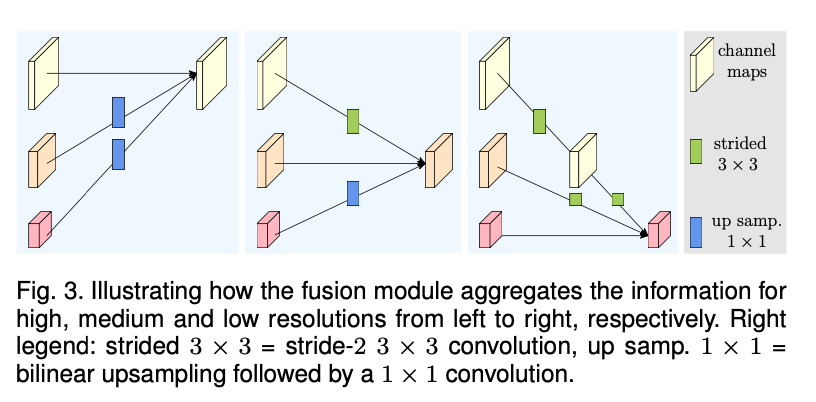Fig3展示了一个融合3种分辨率representation的例子。其可以很容易的扩展到融合2种representation和融合4种representation。在Fig3中，输入包括3种representation：$\{ \mathbf{R}^i_r, r=1,2,3 \}$，$r$是分辨率索引，其对应的输出representation为$\{\mathbf {R}_r^o, r=1,2,3 \}$。每个输出representation都是三个输入representation经过处理后的总和：$\mathbf{R}_r^o=f_{1r}(\mathbf{R}_1^i)+f_{2r}(\mathbf{R}_2^i)+f_{3r}(\mathbf{R}_3^i)$。跨阶段（从阶段3到阶段4）的融合还有一个额外的输出：$\mathbf{R}_4^o=f_{14}(\mathbf{R}_1^i)+f_{24}(\mathbf{R}_2^i)+f_{34}(\mathbf{R}_3^i)$。

$f_{xr}(\cdot)$的形式取决于输入分辨率索引$x$和输出分辨率索引$r$。如果$x=r$，则有$f_{xr}(\mathbf{R})=\mathbf{R}$。如果$x<r$，则$f_{xr}(\mathbf{R})$对输入representation $\mathbf{R}$进行$(r-x)$次步长为2的$3\times 3$卷积来实现下采样。比如，一次步长为2 的$3\times 3$卷积可实现2倍下采样，2个连续的步长为2的$3\times 3$卷积可以实现4倍下采样。如果$x>r$，则$f_{xr}(\mathbf{R})$通过双线性插值进行上采样，并使用$1\times 1$卷积对齐channel的数量。图见Fig3。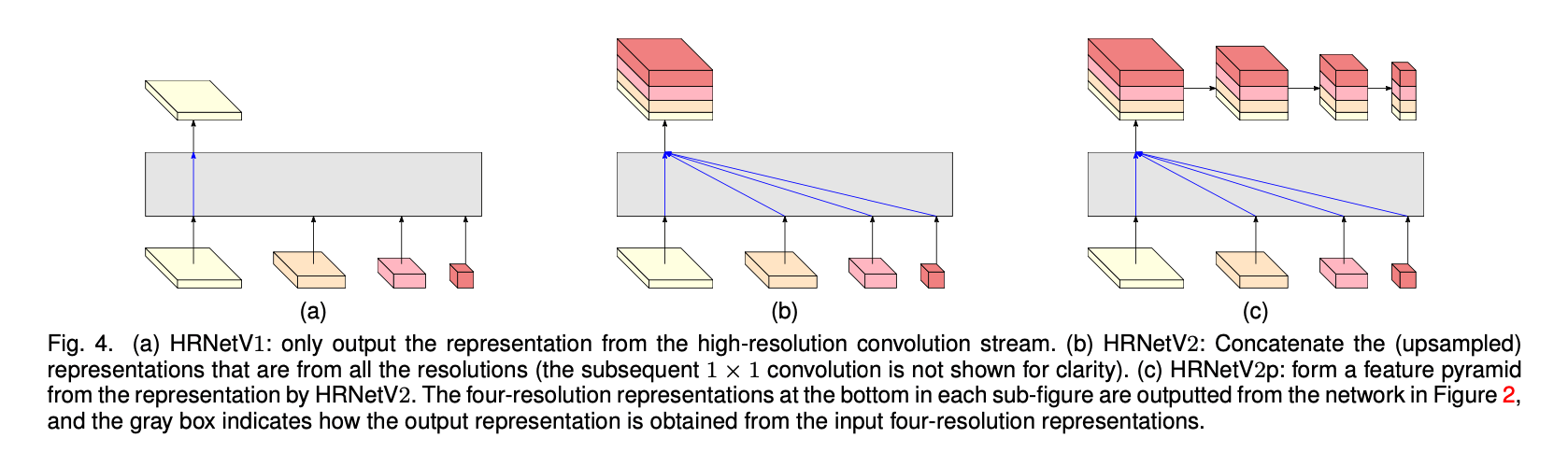👉HRNetV1.

👉HRNetV2.

👉HRNetV2p.

## 3.4.Instantiation

main body包含4个阶段以及4个并行卷积流。4个流的分辨率分别为原始图像的$\frac{1}{4},\frac{1}{8},\frac{1}{16},\frac{1}{32}$。阶段1包括4个residual unit，每个unit都是一个bottleneck的结构。阶段2、阶段3、阶段4分别包括1、4、3个modularized block。每个modularized block都包括4个residual unit。每个unit包括两个$3\times 3$卷积，每个卷积后都接一个BatchNorm和ReLU。

## 3.5.Analysis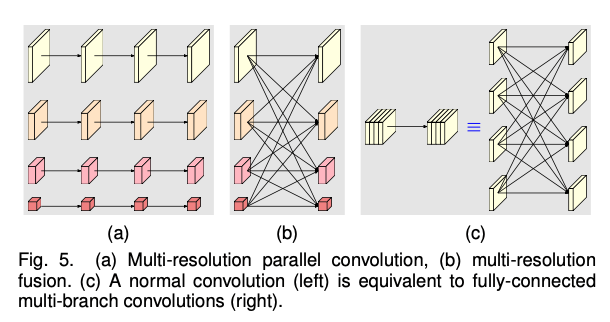# 4.HUMAN POSE ESTIMATION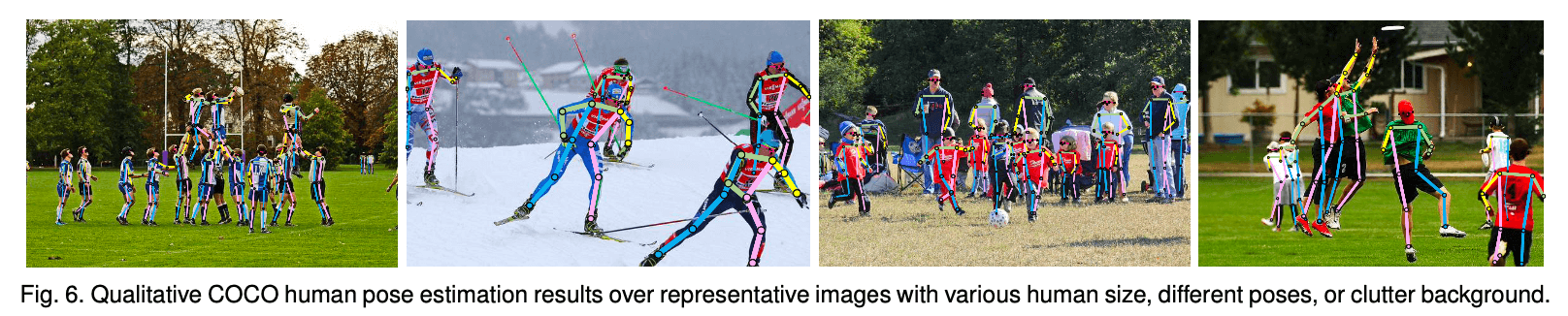Fig6为模型在COCO数据集上的结果，例子包含不同大小的人体、不同姿势以及不同的背景。

👉Dataset.

COCO数据集包含超过200,000张图片，250,000个人物实例，每个人标注有17个关键点。我们在COCO train2017上训练我们的模型，该数据集包含57K张图片和150K个人物实例。我们分别在val2017（包含5000张图片）和test-dev2017（包含20K张图片）上评估我们的模型。

👉Evaluation metric.

$\text{OKS}=\frac{\sum_i \text{exp} [-d^2_i / (2s^2k_i^2)] \delta (v_i>0)}{\sum_i \delta (v_i>0)}$

• $i$表示第$i$个关键点。
• $d_i$为检测到的关键点和GT关键点（即人工标注值）之间的欧氏距离。
• $v_i$表示人工标注关键点是否可见。对于人工标注关键点，$v_i=0$表示关键点未标注，$v_i=1$表示关键点有遮挡但已经标注，$v_i=2$表示关键点无遮挡且已标注。
• $s$为目标尺度（object scale），即此目标在GT中所占面积（比如bounding box的面积）的平方根。
• $k_i$为第$i$个关键点的归一化因子（该因子是所有样本集中关键点由人工标注与真实值存在的标准差，该值越大表示此类型的关键点越难标注）。对COCO数据集中的5000个样本统计出17类关键点的归一化因子：鼻子0.026，眼睛0.025，耳朵0.035，肩膀0.079，手肘0.072，手腕0.062，臀部0.107，膝盖0.087，脚踝0.089。此值可以看作常数，如果使用的关键点类型不在此当中，则需要统计方法计算。
• $\delta$表示如果条件成立则为1，否则为0。

• Average Precision（AP）：
• $\text{AP}$：将第二个阈值分别设置为0.50,0.55,0.60,…,0.90,0.95等10个值，可以算出来10个AP，这10个AP的均值便是最终的AP。
• $\text{AP}^{\text{OKS}=.50}$：第二个阈值设置为0.5时得到的AP。
• $\text{AP}^{\text{OKS}=.75}$：第二个阈值设置为0.75时得到的AP。
• AP Across Scales：
• $\text{AP}^{\text{medium}}$：中等大小的object的AP。这里的中等大小指的是：$32^2 < \text{area} < 96^2$。因为过小的object（$\text{area} < 32^2$）没有标注关键点，所以不考虑。
• $\text{AP}^{\text{large}}$：大obejct的AP，指的是$\text{area}>96^2$的object。
• Average Recall（AR）：
• $\text{AR}$：将第二个阈值分别设置为0.50,0.55,0.60,…,0.90,0.95等10个值，可以算出来10个AR，这10个AR的均值便是最终的AR。
• $\text{AR}^{\text{OKS}=.50}$：第二个阈值设置为0.5时得到的AR。
• $\text{AR}^{\text{OKS}=.75}$：第二个阈值设置为0.75时得到的AR。
• AR Across Scales：
• $\text{AR}^{\text{medium}}$：中等大小（$32^2 < \text{area} < 96^2$）的object的AR。
• $\text{AR}^{\text{large}}$：大obejct的AR，指的是$\text{area}>96^2$的object。

👉Training.

👉Testing.

👉Results on the val set.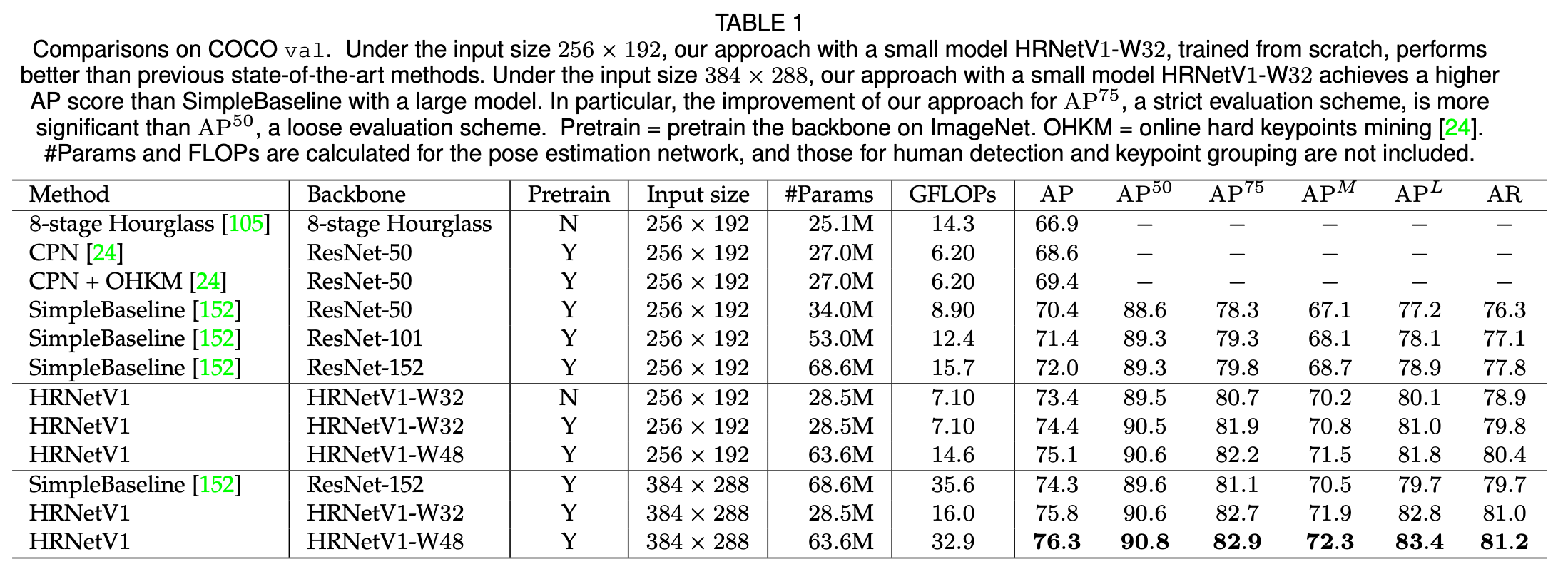👉Results on the test-dev set.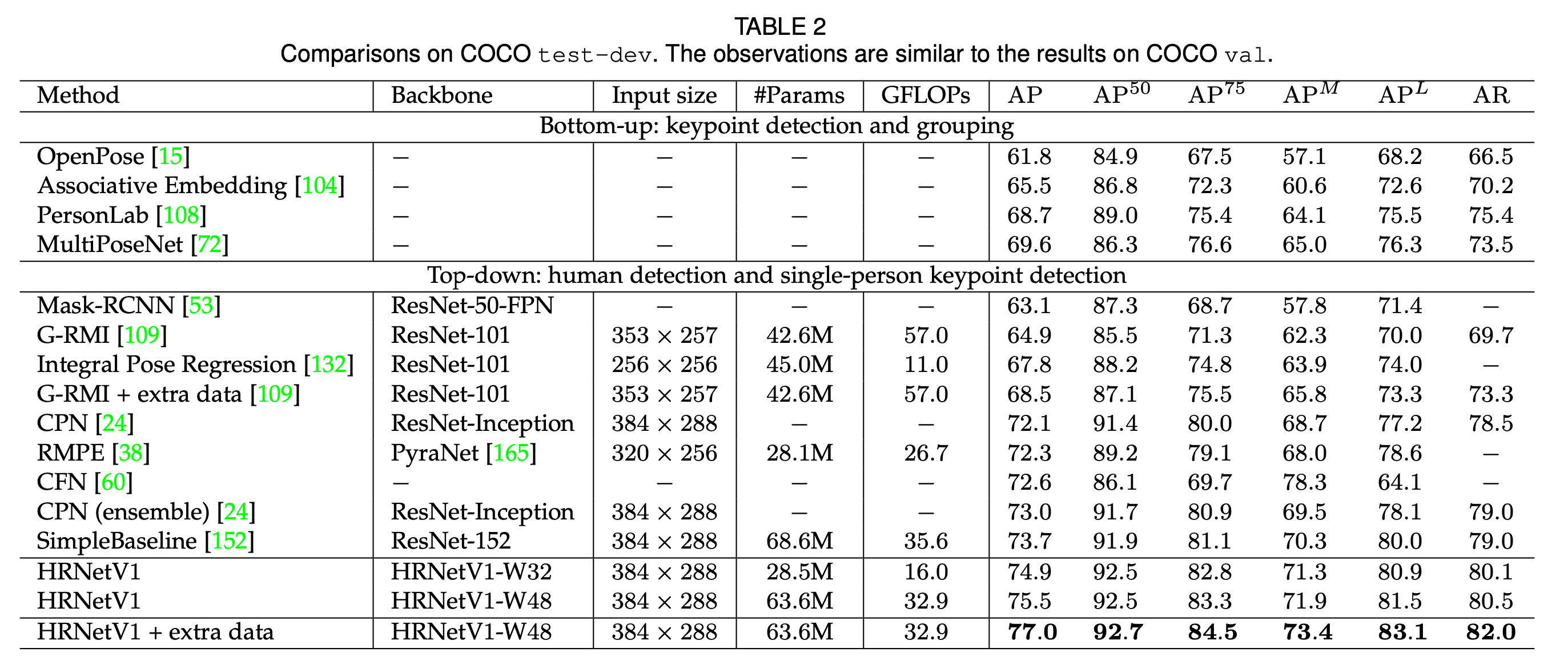# 5.SEMANTIC SEGMENTATION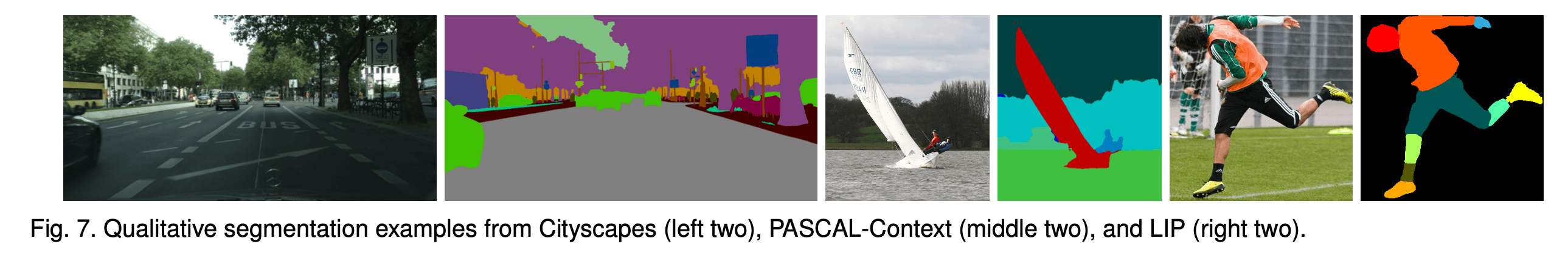👉Cityscapes.

Cityscapes数据集包含5000张像素级别的高质量标注的场景图像。其中训练集2975张图像，验证集500张图像，测试集1525张图像。一共包含30个类别，其中19个类别被用于评估。除了使用mIoU，在测试集中，我们还汇报了另外三个指标的结果：IoU category（cat.）、iIoU class（cla.）以及iIoU category（cat.）。

syncBN（Synchronized Batch Normalization）是一种批量归一化（Batch Normalization，BN）的变种，用于并行训练深度神经网络时加速训练过程和提高模型的性能。

syncBN通过跨多个GPU的所有数据计算每个通道的均值和方差，并将这些值用于所有GPU上的mini-batch数据的标准化。这样可以保证在所有GPU上对数据进行归一化时具有相同的统计特征，从而提高模型的性能和训练速度。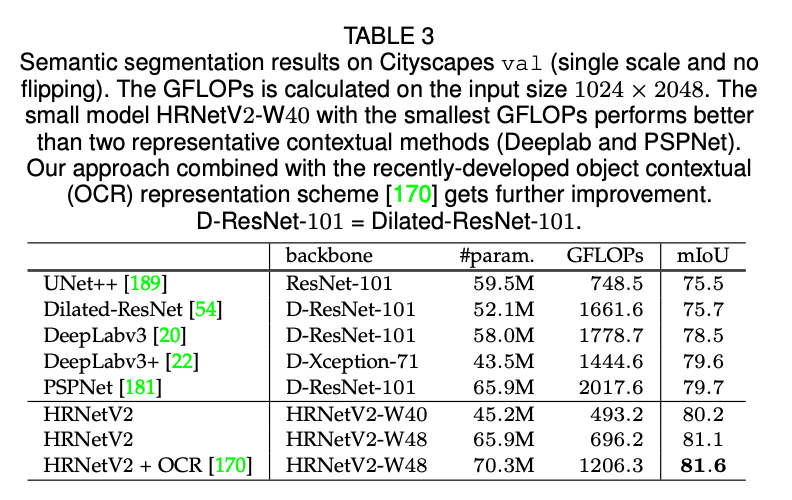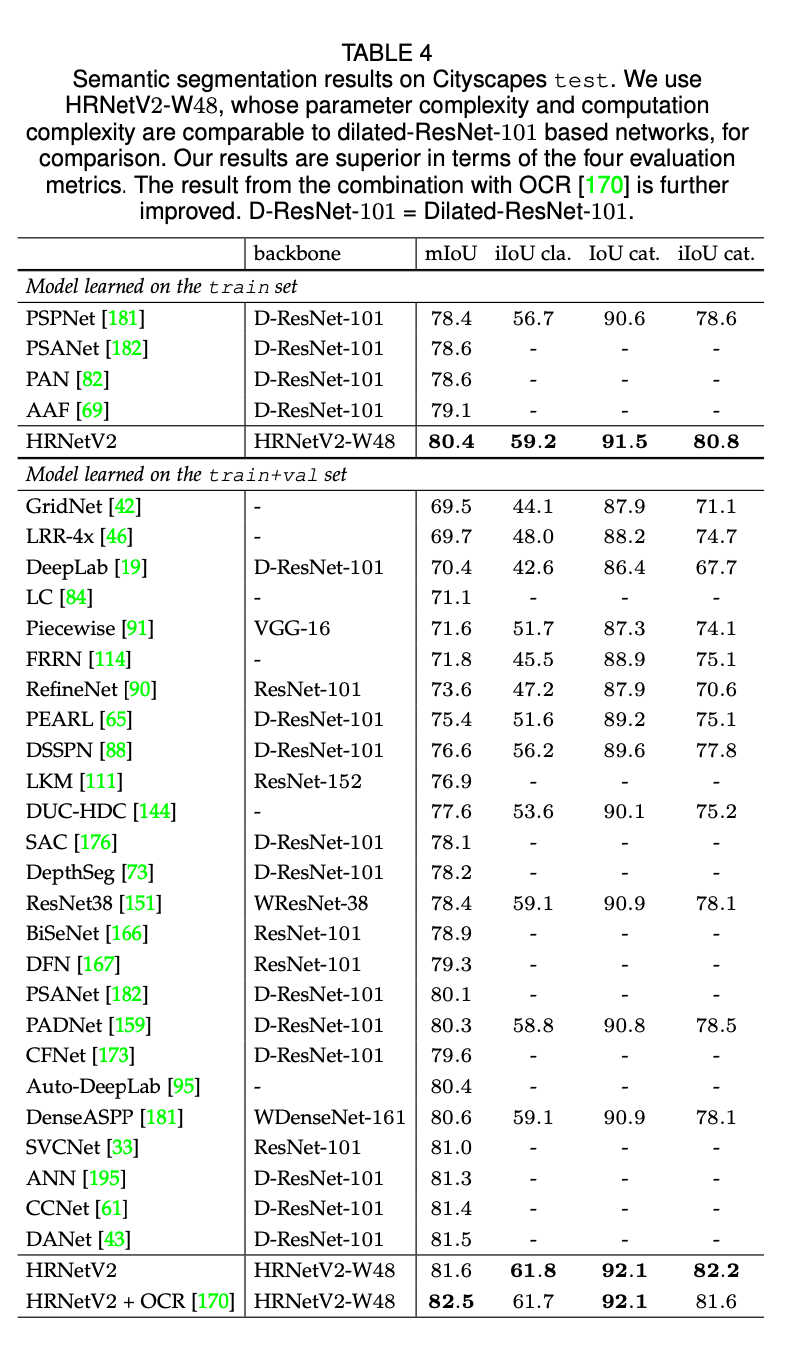👉PASCAL-Context.

PASCAL-Context训练集包括4998张场景图像，测试集包括5105张图像，一共有59个语义标签和一个背景标签。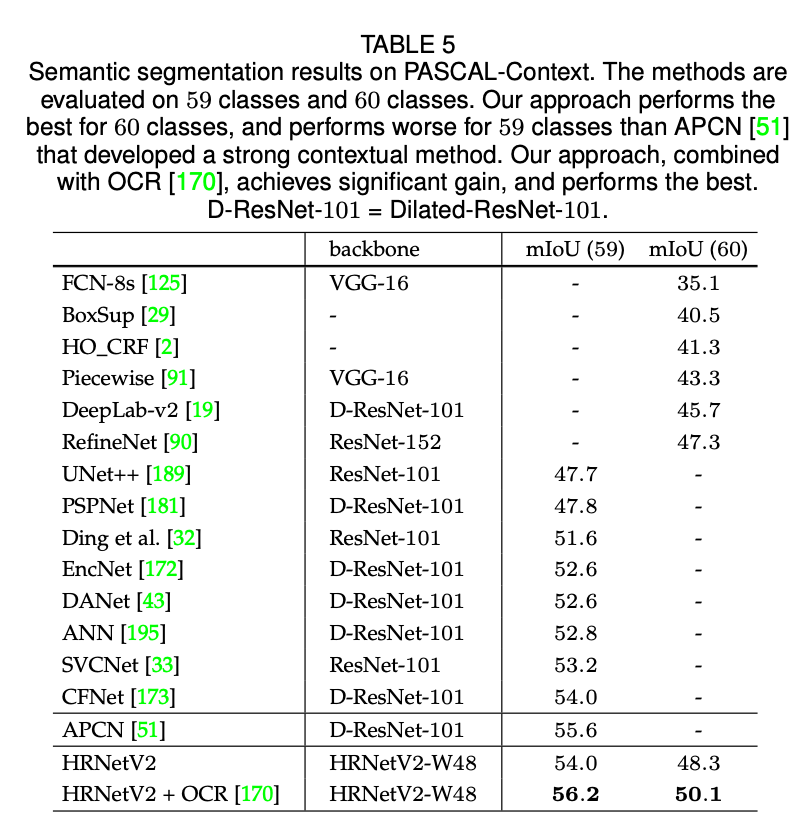👉LIP.

LIP数据集包含50,462张精心标注的人物图像，其中30,462张用于训练，10,000张用于验证。在20个类别（19个人物部位标签+1个背景标签）上进行评估。遵循标准的训练和测试设置，将图像resize到$473 \times 473$，用原始图像和翻转图像结果的平均进行性能评估。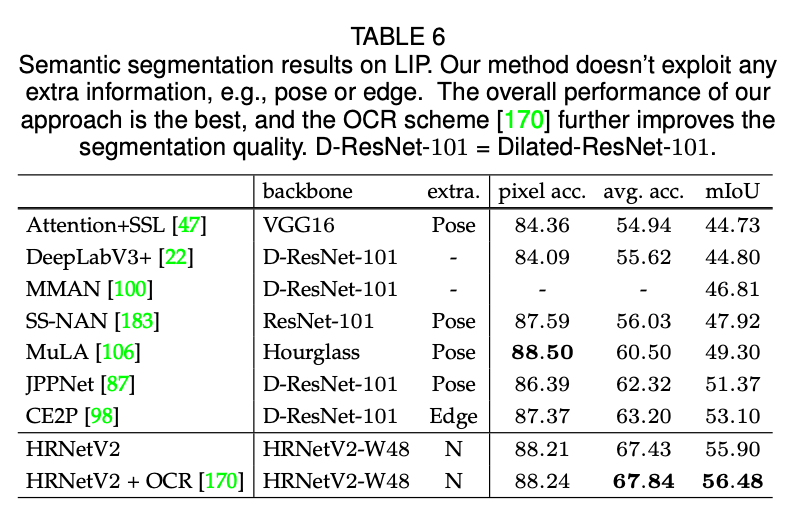# 6.COCO OBJECT DETECTION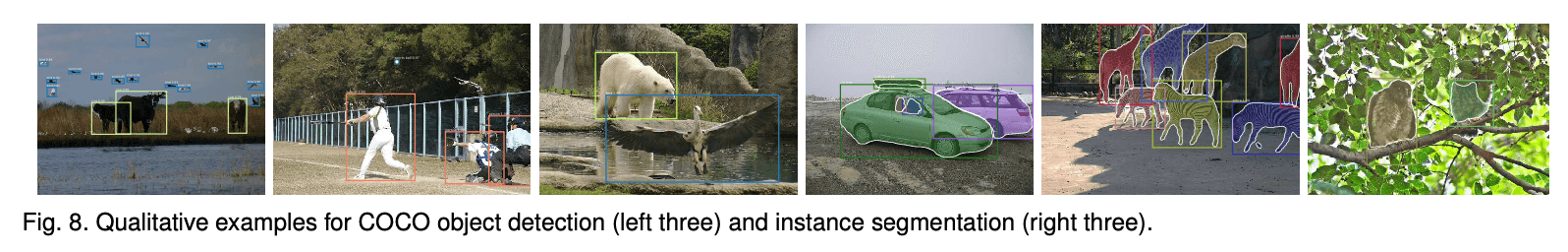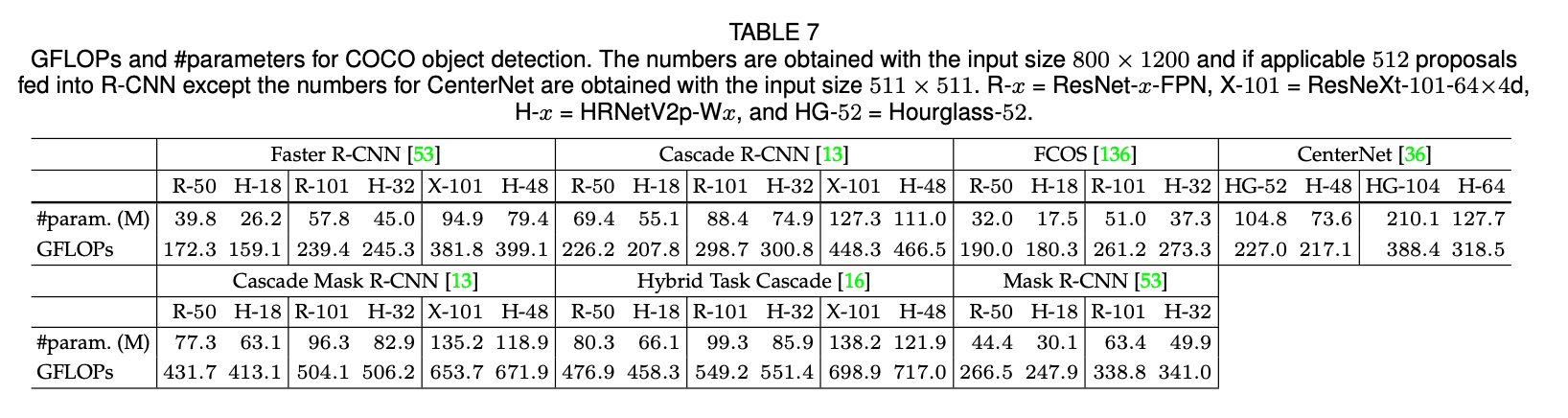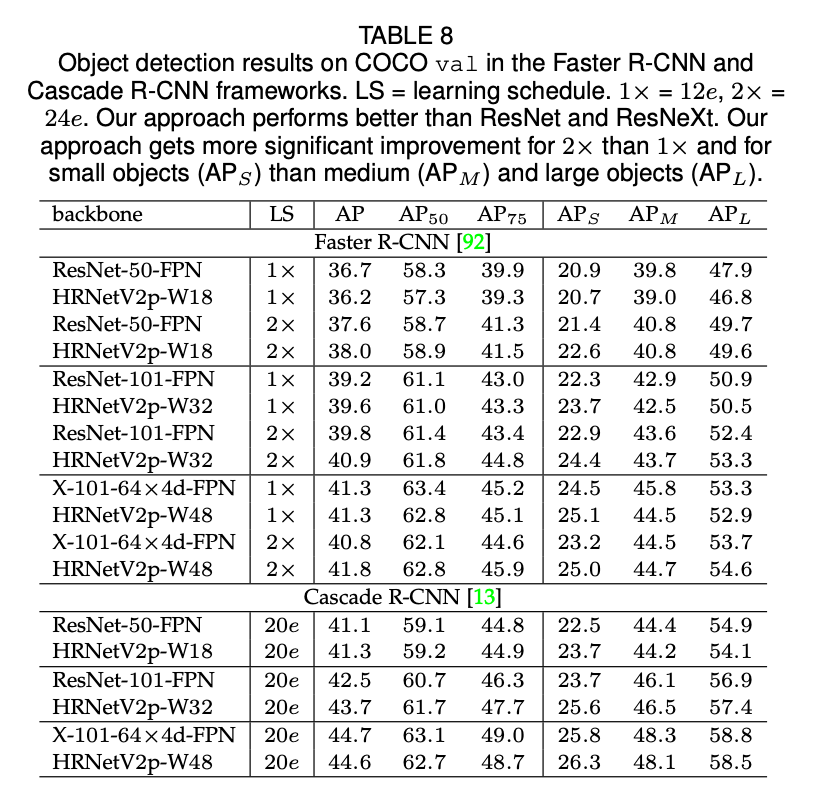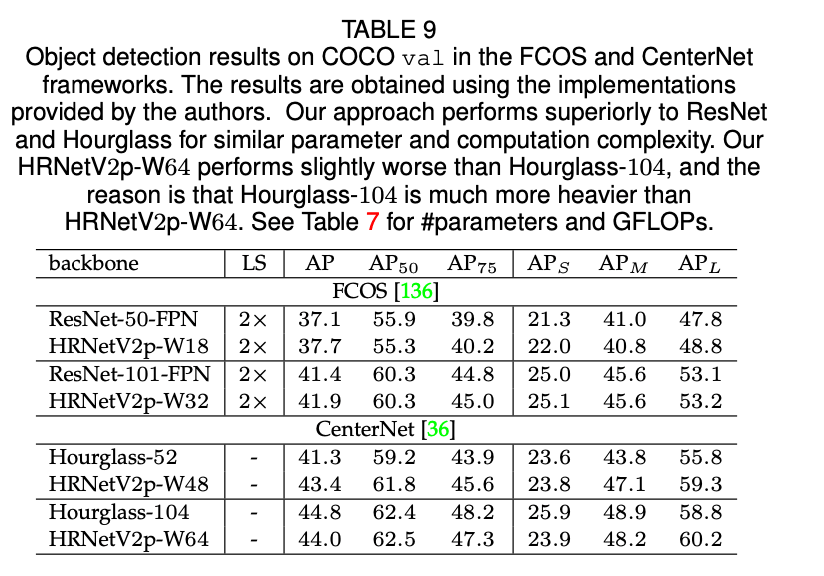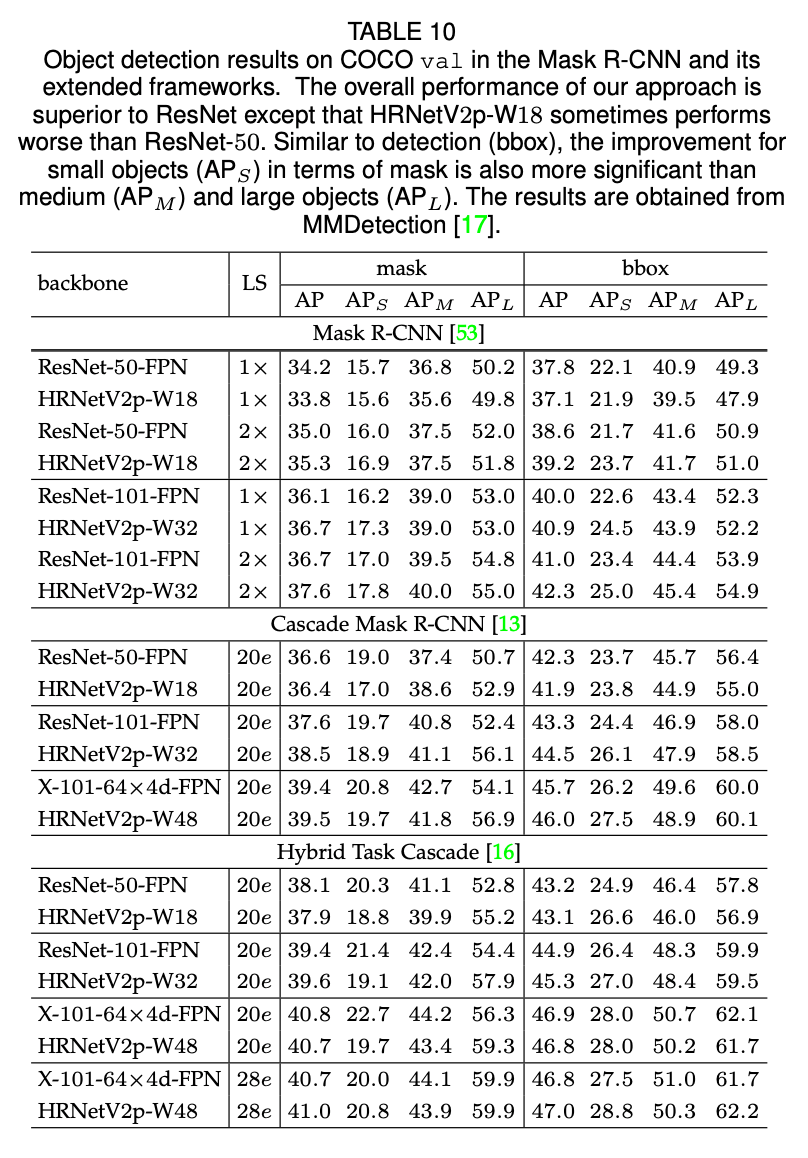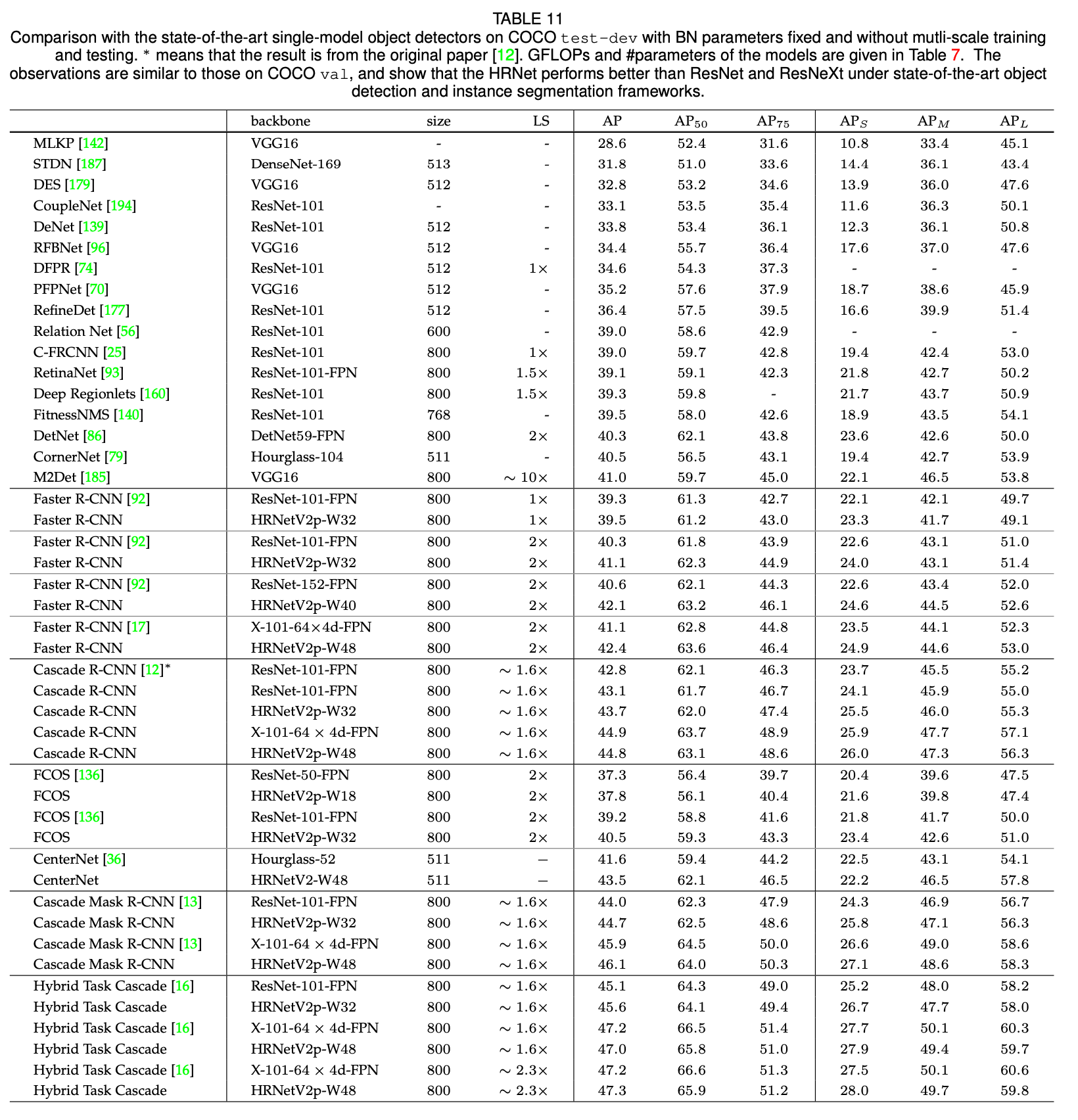# 7.ABLATION STUDY

👉Representations of different resolutions.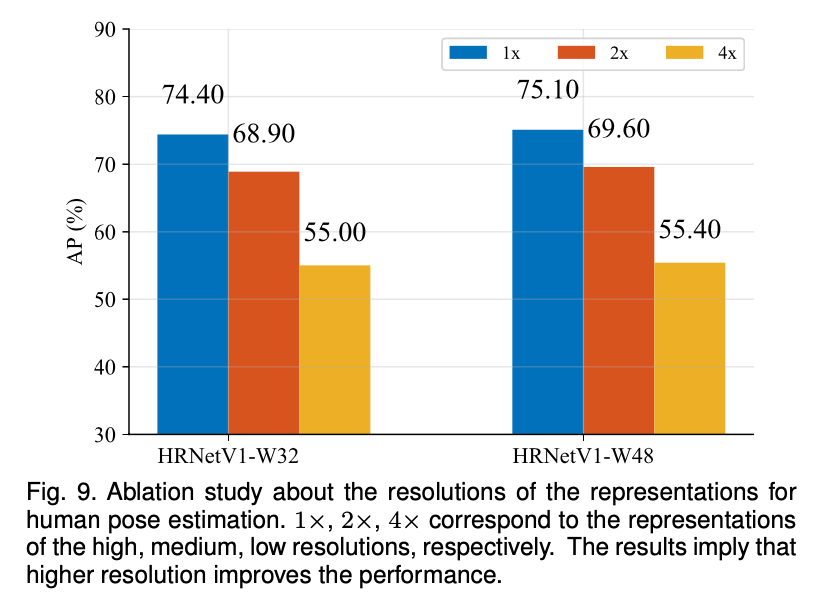👉Repeated multi-resolution fusion.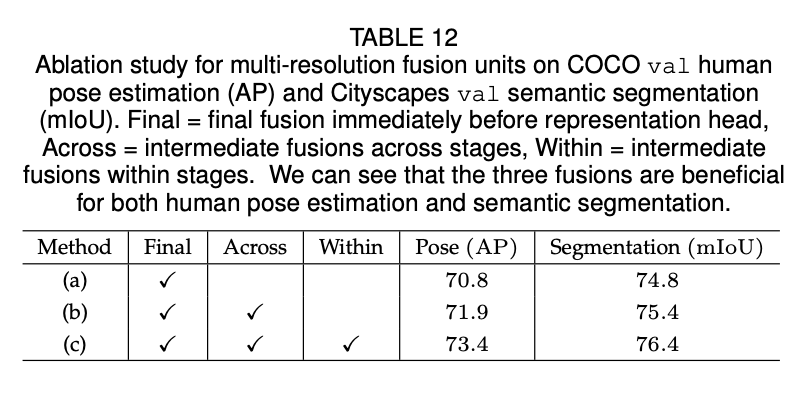👉Resolution maintenance.

V1 vs. V2.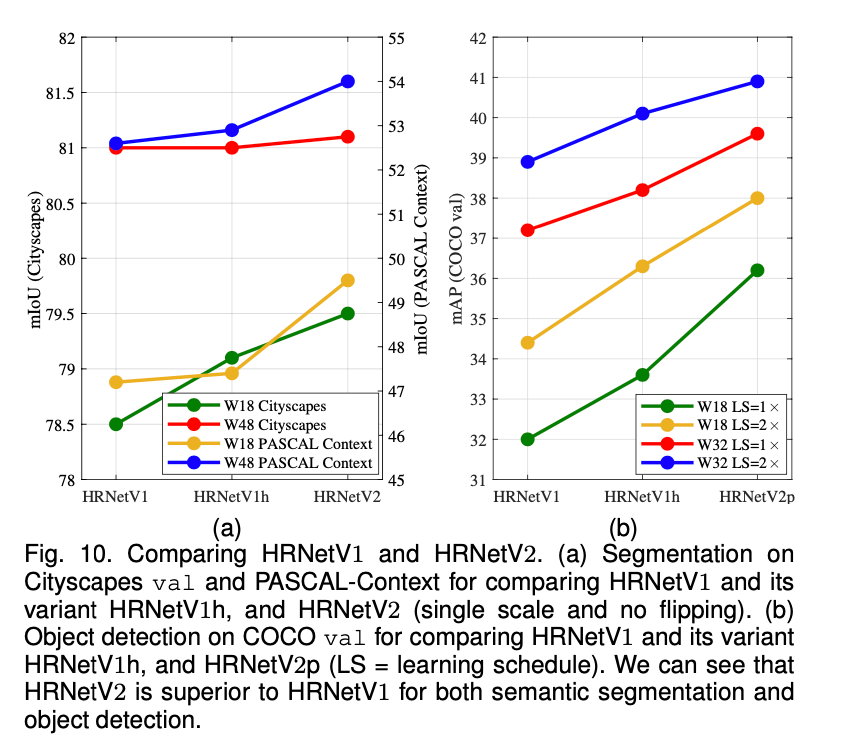# 8.CONCLUSIONS

Discussions.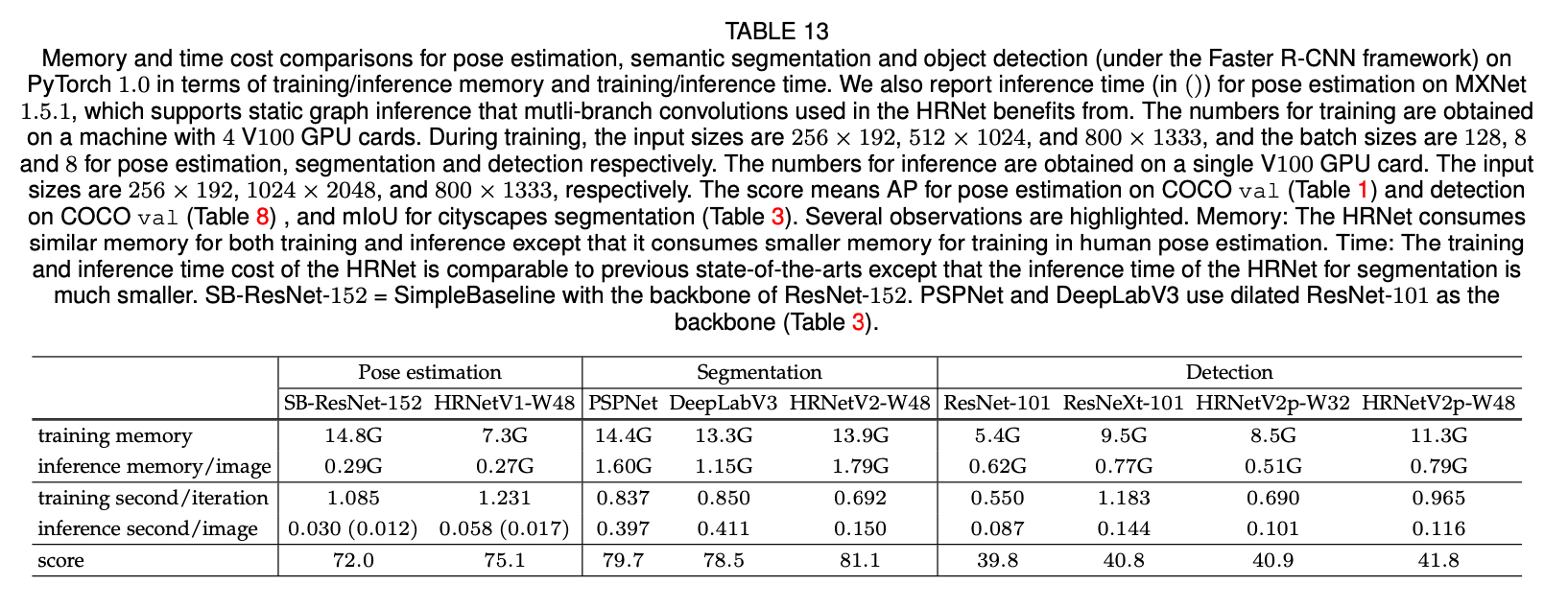Future and followup works.

HRNet的应用并不局限于我们已经列出的，HRNet也适用于其他位置敏感型的视觉应用，比如面部特征点检测、超分辨率、光流估计、深度估计等等。目前已经有了一些后续的工作，比如图像风格化、图像修复、图像增强、图像去雾、时间姿态估计和无人机目标检测等。

# 9.APPENDIX

## APPENDIX A : NETWORK INSTANTIATION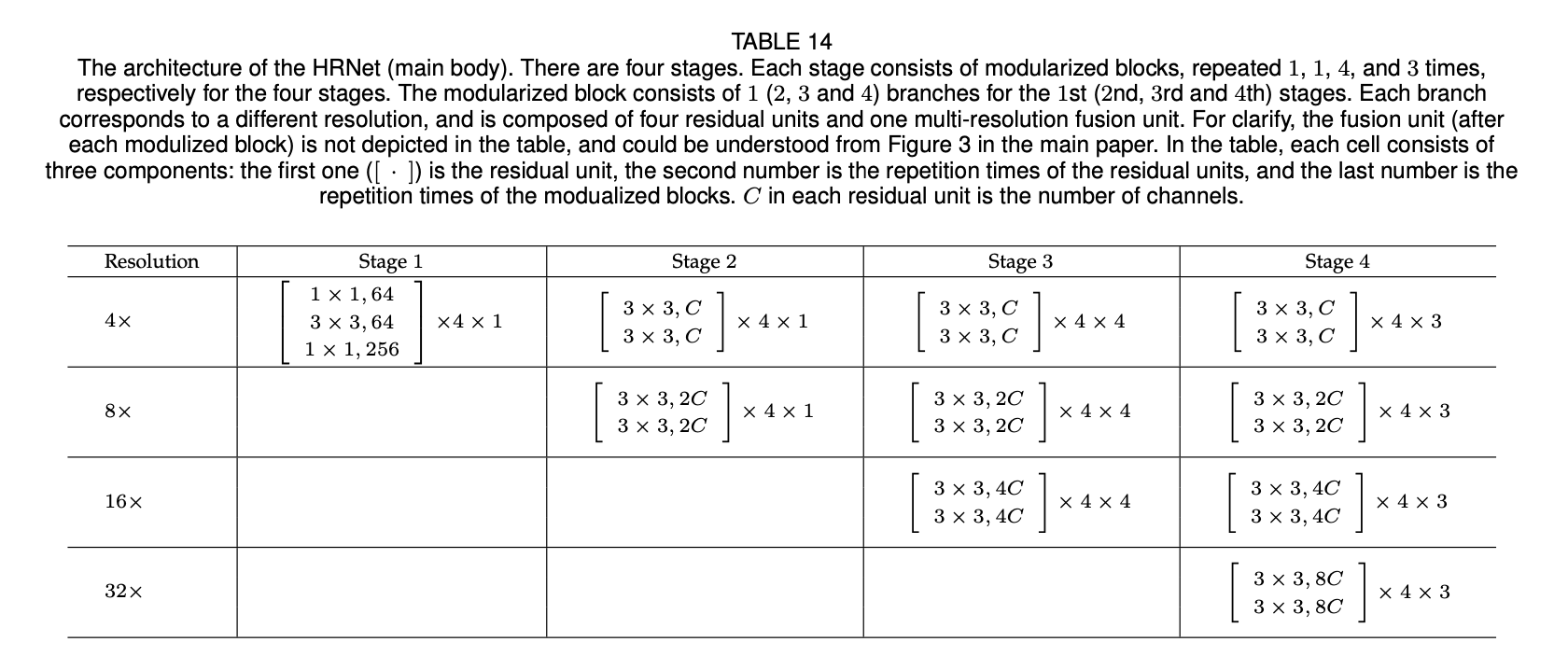## APPENDIX B : NETWORK PRETRAINING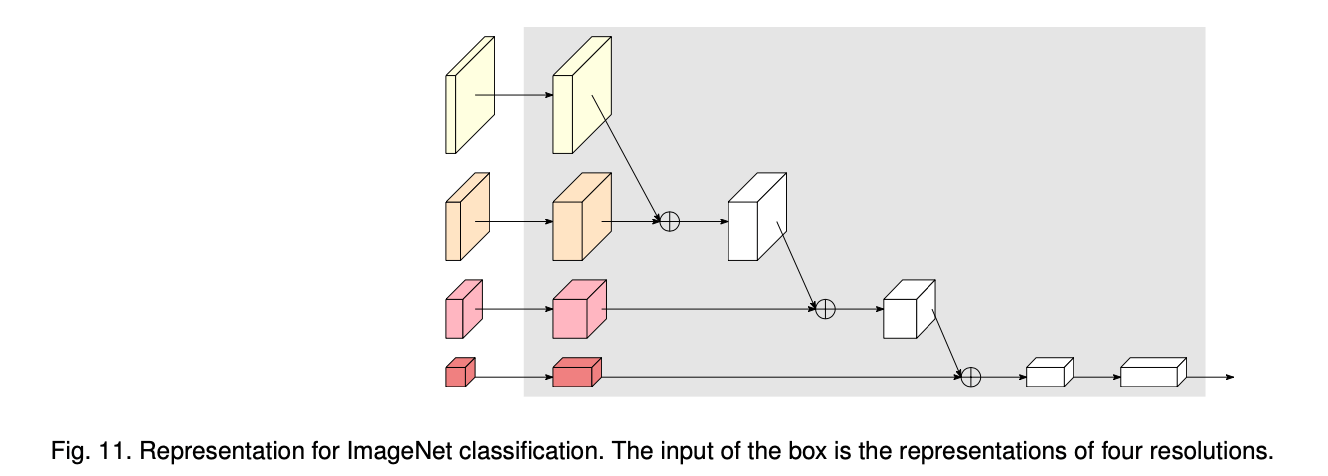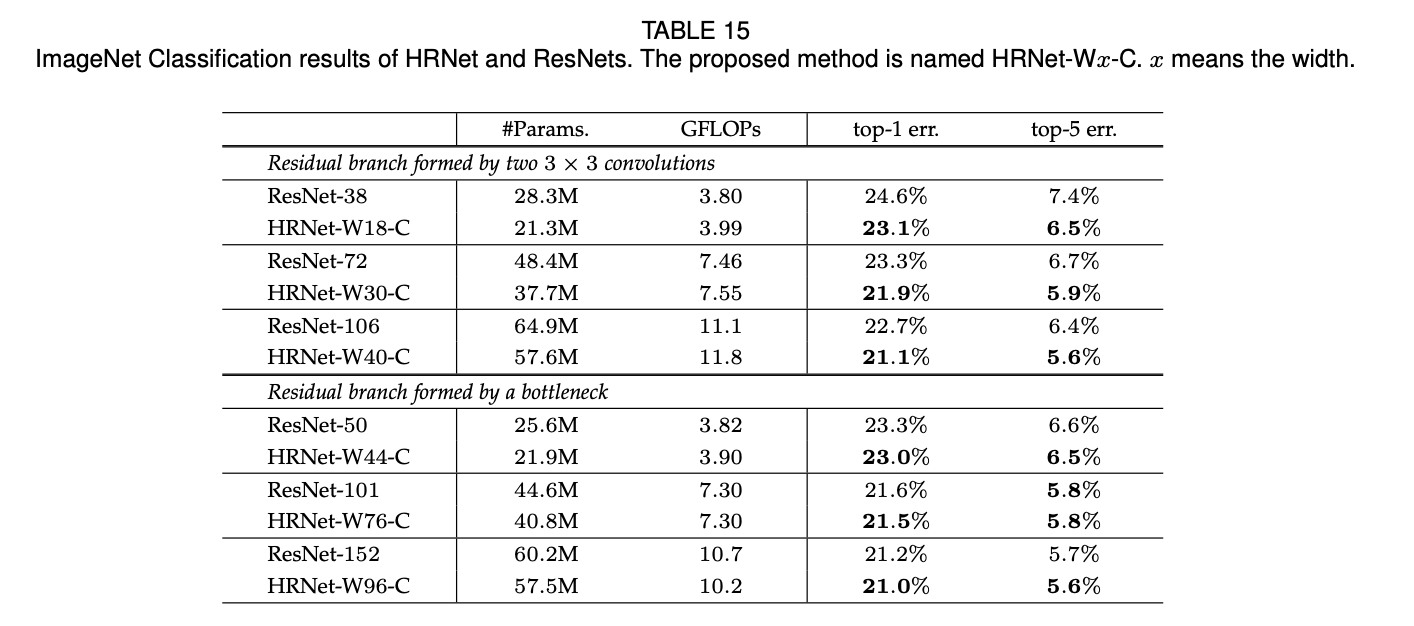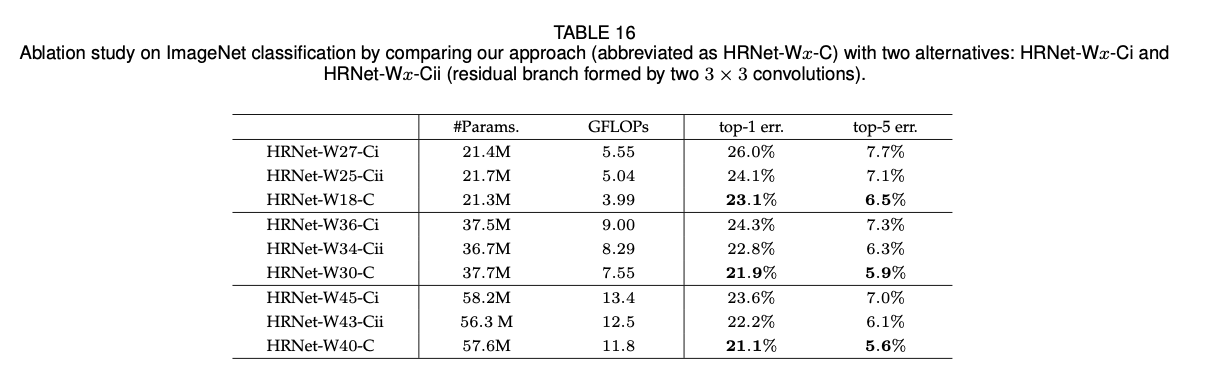## APPENDIX C : TRAINING/INFERENCE COST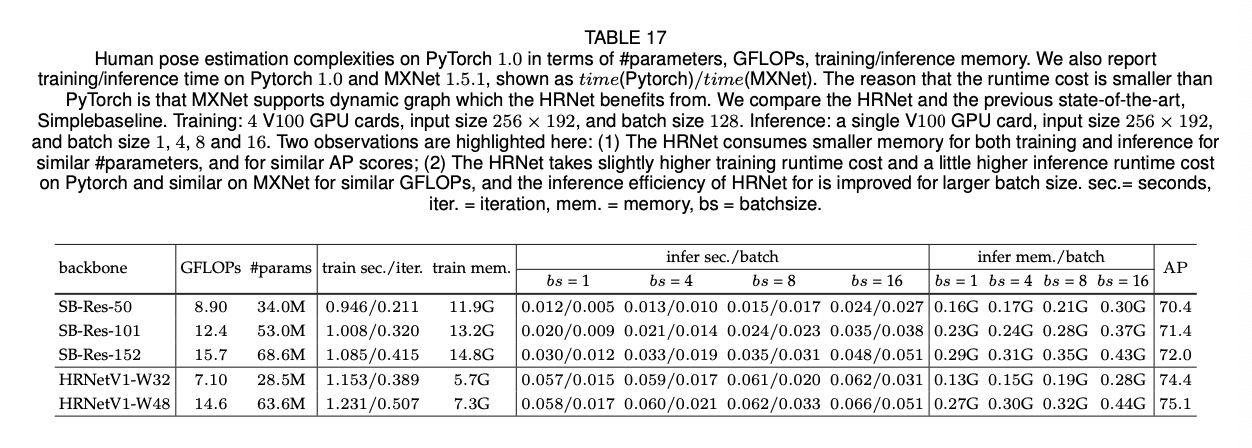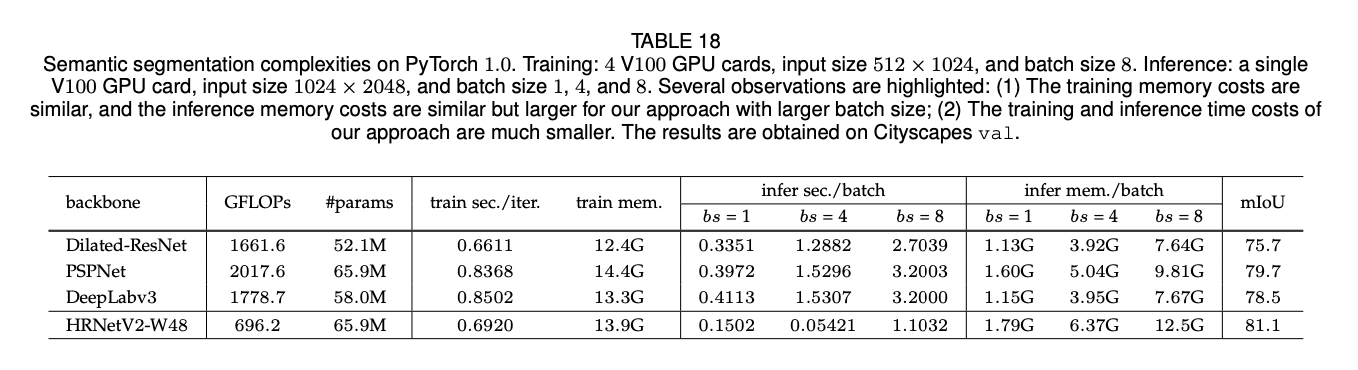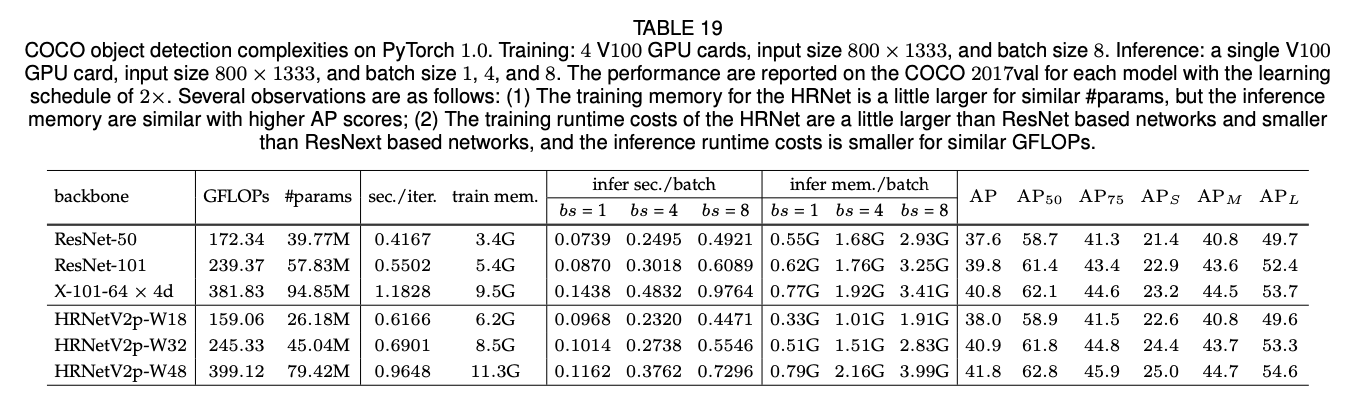## APPENDIX D : FACIAL LANDMARK DETECTION

👉WFLW.

WFLW数据集是最近基于WIDER Face构建的数据集。该数据集包括7500张训练图像和2500张测试图像，每张图像有98个手动标注的关键点。我们在训练集和一些子集上汇报了结果，这些子集包括：large pose（326张图像）、expression（314张图像）、illumination（698张图像）、make-up（206张图像）、occlusion（736张图像）、blur（773张图像）。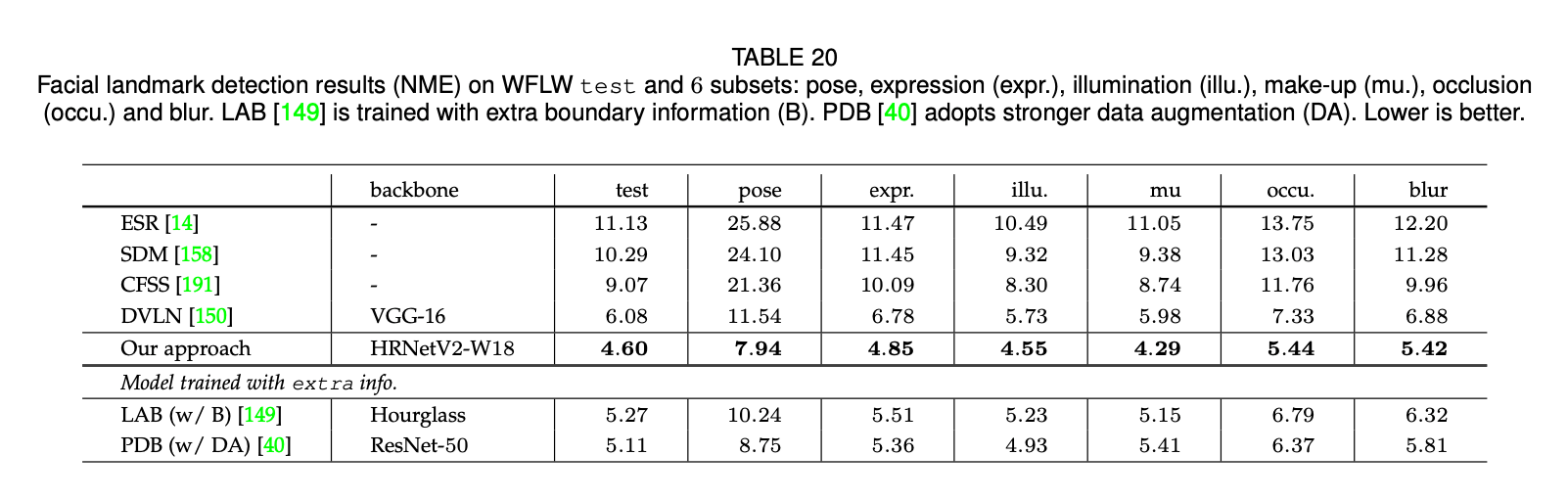👉AFLW.

AFLW数据集是一个广泛使用的benchmark数据集，每张图像包括19个面部关键点。遵循论文“W. Wu, C. Qian, S. Yang, Q. Wang, Y. Cai, and Q. Zhou. Look at boundary: A boundary-aware face alignment algorithm. In CVPR, pages 2129–2138, 2018.”和论文“S. Zhu, C. Li, C. C. Loy, and X. Tang. Face alignment by coarse-to-fine shape searching. In CVPR, pages 4998–5006, 2015.”，我们在20000张训练图像上训练了我们的模型，然后在AFLW-Full数据集（4386张测试图像）和AFLW-Frontal数据集（从4386张测试图像中选出了1314张图像）上汇报了我们的结果。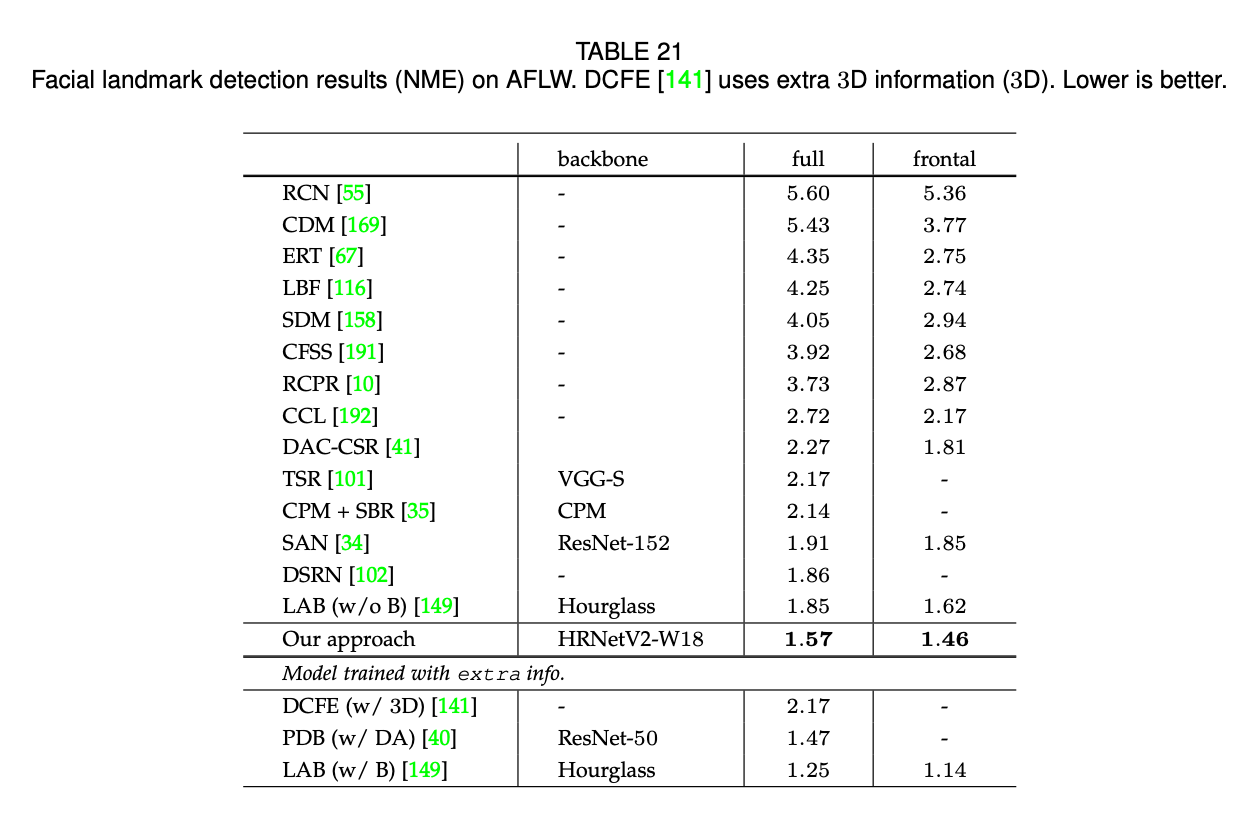👉COFW.

COFW数据集包括1345张训练图像和507张测试图像，图像带有遮挡，每张图像包括29个面部关键点。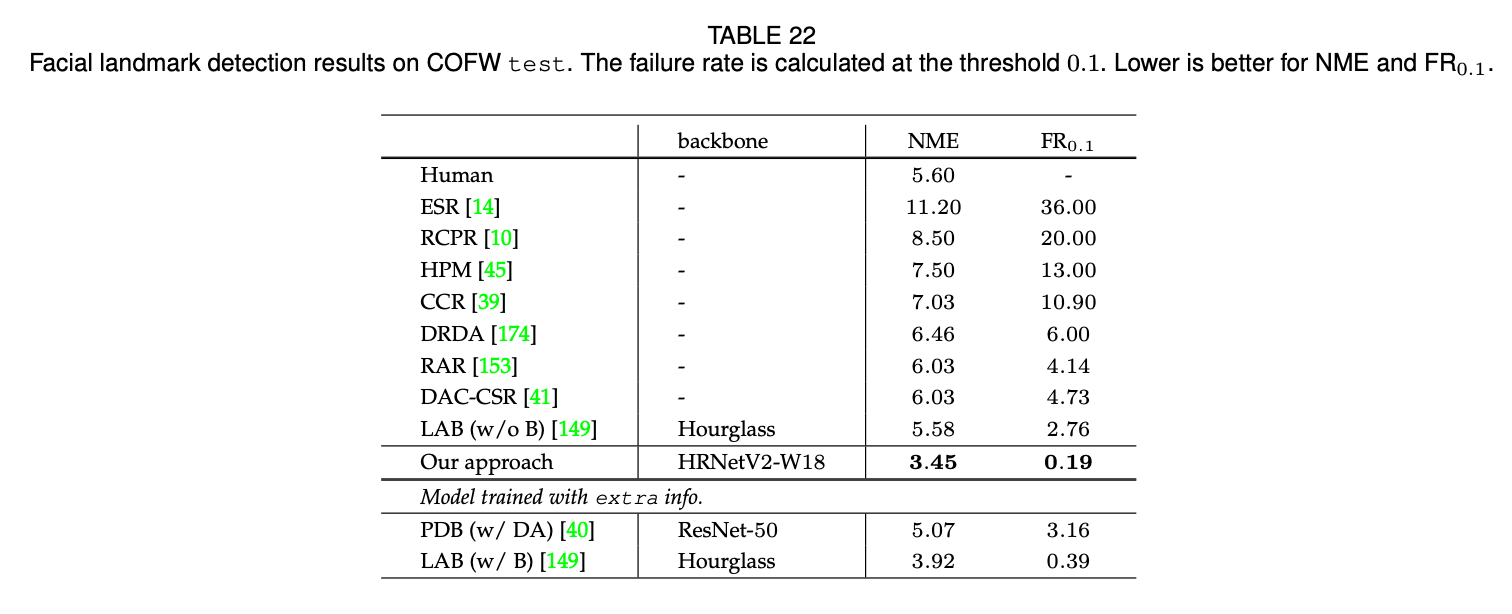👉300W.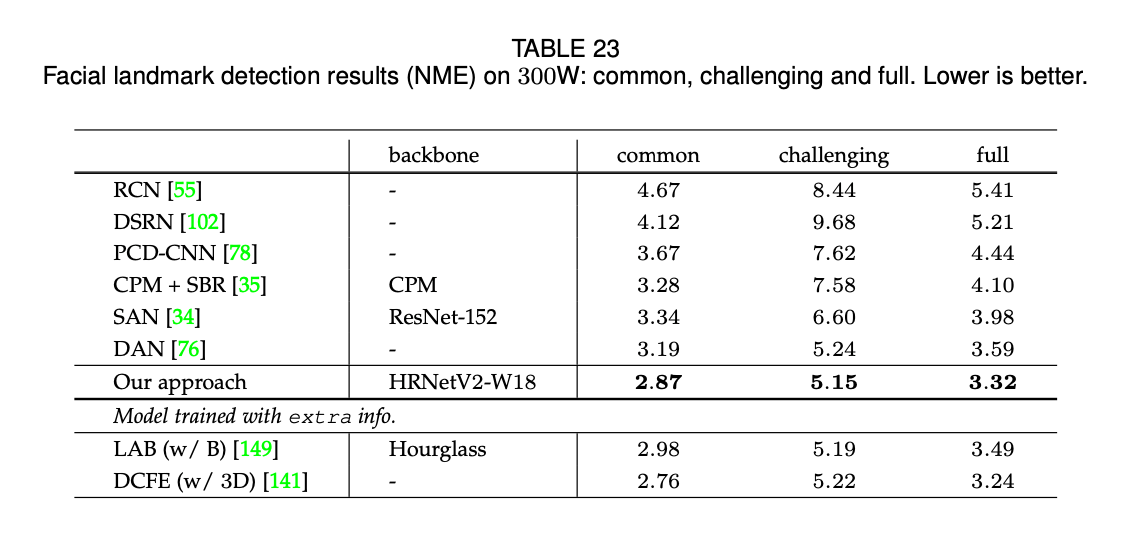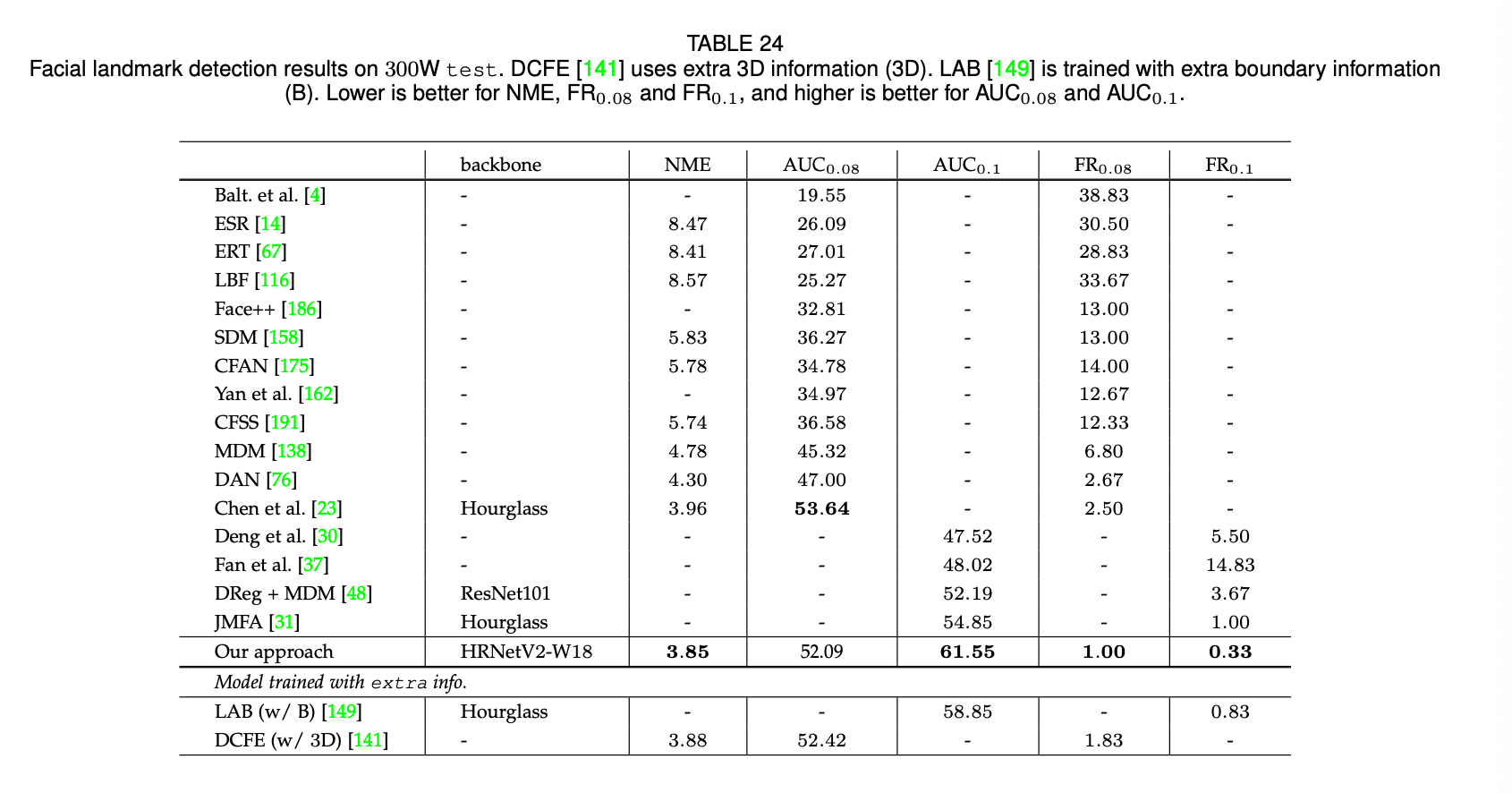## APPENDIX E : MORE OBJECT DETECTION AND INSTANCE RESULTS ON COCO VAL2017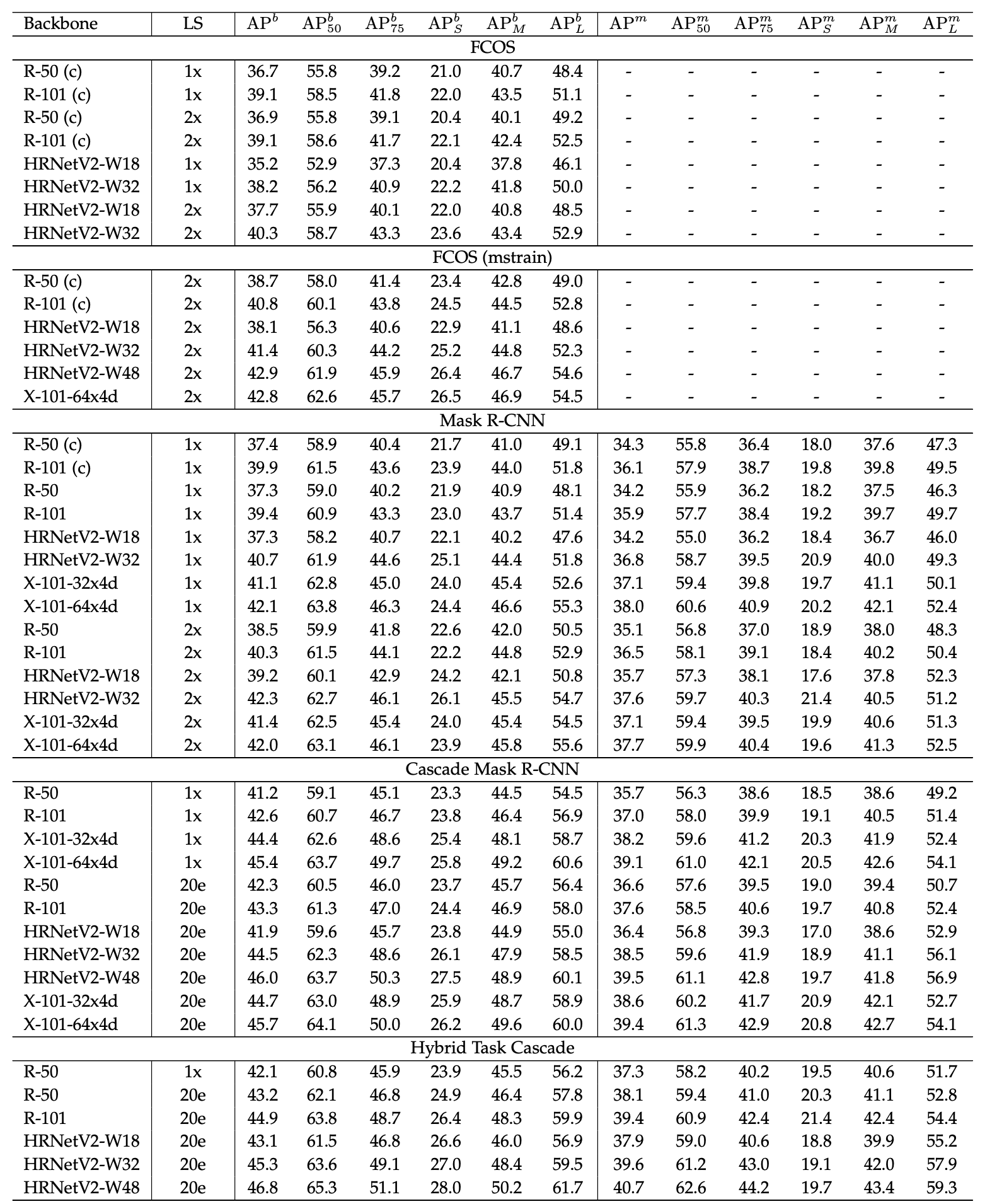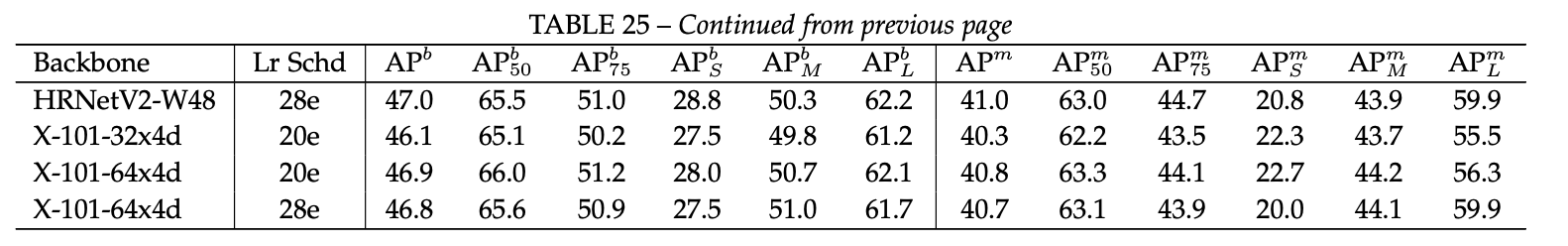# 11.参考资料